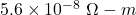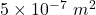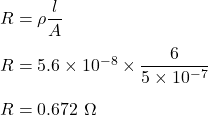Question

What is the resistance of a 6.0 m long gold wire (ρgold = 5.6 x 10 -8 Ω-m) whose cross-sectional area is 5×10 -7m 2 ? (Please show some work)

1.thuthuy

R =0.672 ohms

Explanation:

We have,

Length of a gold wire is 6 m

Resistivity of gold isArea of cross section of the wire isThe resistance of a wire is given by the relation as follows :So, the resistance of the gold wire is 0.672 ohms.